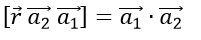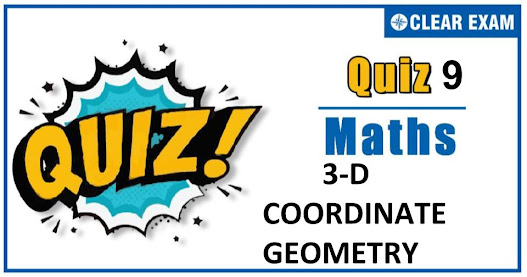## .question { margin-bottom: 20px; border-bottom: 1px solid #ddd; } .ques { font-size: 15px; font-weight: bold; line-height: 150%; } .q-options { padding: 0 !important; list-style: none; margin: 15px 0 !important; } .opt { font-size: 14px; font-weight: normal; } .answer { color: blue; display: none; padding-bottom: 15px; line-height: 150%; } .opt-right.active{ background: #a3ff91; font-size: 19px; line-height: 150%; border: 1px solid ##a3ff91; -webkit-border-radius: 5px; -moz-border-radius: 5px; border-radius: 5px; margin: 10px 0; } .mcq-info { margin: 20px 0 40px; color: #060553; line-height: 150%; } .timer{ overflow: hidden; margin: 20px 0; background: #f7f7f7; padding: 10px; border: 1px solid #eee; border-radius: 3px; } .start-btn, .time-btn, .stop-btn, #reset-start{ border: 1px solid #777; -o-border-radius: 2px; -ms-border-radius: 2px; -webkit-border-radius: 2px; border-radius: 2px; color: #fff; padding: 7px 15px; width: auto; height: auto; line-height: 150%; background: #d12F2F; cursor: pointer; margin-right: 10px; } #reset-start{ background: #0E2230; color: #fff; border-color: #0E2230; display: none; } .start-btn:hover, .time-btn:hover, .stop-btn:hover{ background: #0E2230; color: #fff; border-color: #0E2230; } #reset-start:hover{ background: #d12F2F; color: #fff; border-color: #d12F2F; } @media screen and (max-width: 767px){ .ques, .answer, .mcq-info, .opt{ font-size: 13px; } } Q1. The distance between the points (1,4,5) and (2,2,3) is  2  5  4  3 Solution The distance between given points =√((2-1)^2+(2-4)^2+(3-5)^2 ) =√(1+4+4)=3 Q2.The distance of the point of intersection of the line (x-2)/3=(y+1)/4=(z-2)/12 and the plane x-y+z=5 from the point (-1,-5,-10)is  8  13  12  11 Solution Given line is (x-2)/3=(y+1)/4=(z-2)/12=k (say) Any point on the line is (3k+2,4k-1,12k+2) This point lies on the plane x-y+z=5 ∴3k+2-(4k-1)+12k+2=5 ⟹11k=0 ⟹k=0 ∴ Intersection point is (2,-1,2) ∴ Distance, between points(2-1,2) and (-1,-5,-10) =√((-1-2)^2+(-5+1)^2+(-10-2)^2 ) =√(9+16+144)=13 Q3.  The triangle formed by the points (0, 7, 10), (-1,6,6),(-4,9,6) is   Equilateral  Isosceles  Right angled  Right angled isosceles Solution Let A(0,7,10),B(-1,6,6) and C(-4,9,6) Then, AB=√((-1-0)^2+(6-7)^2+(6-10)^2 ) =√(1+1+16)=√18=3√2 BC=√((-4+1)^2+(9-6)^2+(6-6)^2 ) =√(9+9+0)=√18=3√2 AC=√((-4-0)^2+(9-7)^2+(6-10)^2 ) =√(16+4+16)=√36=6 Clearly, AC^2=AB^2+BC^2 Hence, triangle is right angled. Also, AB=BC ∴ Triangle is right angled isosceles Q4. The equation of the plane containing the lines r ⃗=(a1 ) ⃗+λ (a2 ) ⃗ and r ⃗=(a2 ) ⃗+λ (a1 ) ⃗, isNone of these  [r ⃗ (a_1 ) ⃗ (a_2 ) ⃗ ]=(a_1 ) ⃗∙(a_2 ) ⃗  [r ⃗ (a_1 ) ⃗ (a_2 ) ⃗ ]=0 SolutionQ5.The lines r ⃗=a ⃗+λ(b ⃗×c ⃗ ) and r ⃗=b ⃗+μ(c ⃗×a ⃗ ) will intersect, if  a ⃗∙c ⃗=b ⃗∙c ⃗  a ⃗×c ⃗=b ⃗×c ⃗  b ⃗×a ⃗=c ⃗×a ⃗  None of these Solution  The lines r ⃗=a ⃗+λ(b ⃗×c ⃗ ) and r ⃗=b ⃗+μ(c ⃗×a ⃗ ) pass through points a ⃗ and b ⃗ respectively and are parallel to vectors b ⃗×c ⃗ and c ⃗×a ⃗ respectively. Therefore, they will intersect, if a ⃗-b ⃗,b ⃗×c ⃗ and c ⃗×a ⃗ are coplanar ⇒(a ⃗-b ⃗ )∙{(b ⃗×c ⃗ )×(c ⃗×a ⃗ )}=0 ⇒(a ⃗-b ⃗ )∙{[b ⃗ c ⃗ a ⃗ ] c ⃗-[b ⃗ c ⃗ c ⃗ ] a ⃗ }=0 ⇒(a ⃗-b ⃗ )∙c ⃗[b ⃗ c ⃗ a ⃗ ]=0 ⇒a ⃗∙c ⃗-b ⃗∙c ⃗=0⇒a ⃗∙c ⃗=b ⃗∙c ⃗ Q6. The equation of the plane passing through the origin and containing the line (x-1)/5=(y-2)/4=(z-3)/5 is  x+5y-3z=0  x-5y+3z=0 x-5y-3z=0  3x-10y+5z=0 Solution The equation of the plane through given line is a(x-1)+b(y-2)+c(z-3)=0 …(i) Since, the straight line lie on the plane. ∴ DR’s of the plane is perpendicular to the line ie, 5a+4b+5c=0 …(ii) Since, the plane passes through (0, 0, 0), we get -a-2b-3c=0 ⟹a+2b+3c=0 ….(iii) On solving Eqs. (ii) and (ii), we get a/2=b/(-10)=c/6 From Eq. (i), 2(x-1)-10(y-2)+6(z-3)=0 ⟹2x-10y+6z=0 ⟹x-5y+3z=0 Q7.If the plane 3x+y+2z+6=0 is parallel to the line (3x-1)/2b=3-y=(z-1)/a, then the value of 3a+3b is  3  1/2  3/2  4 Solution Given line can be rewritten as (x-1/3)/(2b/3)=(y-3)/(-1)=(z-1)/a Given plane3x+y+2z+6=0 is parallel to the above line ∴2b/3∙3+1∙(-1)+2∙a=0 ⟹2a+2b=1 ⟹3a+3b=3/2 Q8.If the direction cosines of two lines are such that l+m+n=0,l^2+m^2+n^2=0, then the angle between them is  π  π/3  π/4  π/6 Solution Given, l+m+n=0 ….(i) and l^2+m^2-n^2=0 …..(ii) ∴ l^2+m^2-(-l-m)^2=0 ⟹2lm=0 ⟹l=0 or m=0 if l=0,then n=-m ⟹l:m:n=0:1:-1 and if m=0, then n=-1 ⟹l:m:n=1:0:-1 ∴cos⁡〖θ=(0+0+1)/(√(0+1+1) √(0+1+1))=1/2〗 ⟹ θ=π/3 Q9.The equation of the plane through the point (1, 2,3) and parallel to the plane x+2y+5z=0 is  x+2y+5z=6  None of the above  x+2y+5z=14  (x-1)+2(y-2)+5(z-3)=0 Solution Equation of plane passing through the point (1, 2, 3) is A(x-1)+B(y-2)+C(z-3)=0 …(i) Since, plane (i) is parallel to plane x+2y+5z=0 ⇒A=1,B=2,C=5 Putting these values in Eq. (i), we get (x-1)+2(y-2)+5(z-3)=0 is the required plane Q10. The line drown from (4,-1,2) the point (-3,2,3) meets a plane at right angle at the point (-10,5,4), then the equation of plane is  7x+3y+z+89=0  7x-3y-z+89=0  7x-3y+z+89=0 None of these Solution Since, the line passing through the points (4,-1,2) and (-3,2,3). So, the DR’s of the line is (4+3,-1-2,2-3)ie,(7,-3,-1) Since, the line is perpendicular to the plane therefore DR’s of this line is proportional to the normal of the plane. ∴ Required equation plane is 7(x+10)-3(y-5)-1(z-4)=0 ⟹7x-3y-z+89=0 var answers = ["D","B","D","D","A","B","C","B","D","B"]; var tot = answers.length; function getCheckedValue( radioName ){ var radios = document.getElementsByName( radioName ); //console.log(radios) for (var y=0; y < radios.length; y++){ if (radios[y].checked) return radios[y].value; } } function getScore(){ var score = 0; for (var i=0; i<tot; i++){ var answerVal = getCheckedValue('question'+i) //console.log(answerVal, answers[i]) if (typeof answerVal != undefined && answerVal == answers[i]) score += 1; } return score; } function returnScore(){ alert('Your score is' +getScore()+"/"+tot); } var q, m, s; function quizCount(){ if(!q){ console.log('mobile2'); m = 1, s = 0; } if(q){ clearTimeout(q); } m = 10, s = 0; \$('#timer').val(m + ":" + s); s = s-1; console.log(s) q = setTimeout("counter()", 1000); if(s<1){ m = m-1; console.log(m) s = 59; } } function counter(){ console.log(m); \$('#timer').val(m + ":" + s); s = s-1; console.log(s) q = setTimeout("counter()", 1000); if(s<1){ m = m-1; console.log(m) s = 59; } if(m<0){ quizStop(); } } function quizStop(){ clearTimeout(q); q= null; m = null; s = null; document.getElementById('timer').value= 'Quiz Finished'; alert("Your score is "+ getScore() +"/"+ tot); \$('.answer').slideDown(); \$('.opt-right').addClass('active'); \$('.start-btn, #reset').hide(); \$('#reset-start').show(); } function quizReset(){ clearTimeout(q); document.getElementById('timer').reset(); } \$(document).ready(function(){ // var a = \$('.question .q-options li .rad').length; \$('.submit').click(function(){ returnScore(); \$('.opt-right').addClass('active'); \$('.answer').slideDown(); }); \$('#reset').click(function(){ quizReset(); }); \$('#reset-start').click(function(){ \$('.opt-right').removeClass('active'); \$('.answer').slideUp(); \$('.start-btn, #reset').show(); \$('#reset-start').hide(); \$('.rad').prop('checked', false); }); });#### Written by: AUTHORNAME

AUTHORDESCRIPTION## Want to know more

Please fill in the details below:

## Latest NEET Articles\$type=three\$c=3\$author=hide\$comment=hide\$rm=hide\$date=hide\$snippet=hide

Name

ltr
item
BEST NEET COACHING CENTER | BEST IIT JEE COACHING INSTITUTE | BEST NEET & IIT JEE COACHING: 3-D COORDINATE GEOMETRY QUIZ-9
3-D COORDINATE GEOMETRY QUIZ-9
https://1.bp.blogspot.com/-TwzA3BJd_bI/X4CWVivAjJI/AAAAAAAABnQ/U7WAWKs3DlgEwDvrrJU31or_2VCG8jIRQCLcBGAsYHQ/w527-h325/Quiz%2BImage%2BTemplate%2B%25286%2529.jpg
https://1.bp.blogspot.com/-TwzA3BJd_bI/X4CWVivAjJI/AAAAAAAABnQ/U7WAWKs3DlgEwDvrrJU31or_2VCG8jIRQCLcBGAsYHQ/s72-w527-c-h325/Quiz%2BImage%2BTemplate%2B%25286%2529.jpg
BEST NEET COACHING CENTER | BEST IIT JEE COACHING INSTITUTE | BEST NEET & IIT JEE COACHING
https://www.cleariitmedical.com/2020/10/3-d-coordinate-geometry-quiz-9.html
https://www.cleariitmedical.com/
https://www.cleariitmedical.com/
https://www.cleariitmedical.com/2020/10/3-d-coordinate-geometry-quiz-9.html
true
7783647550433378923
UTF-8